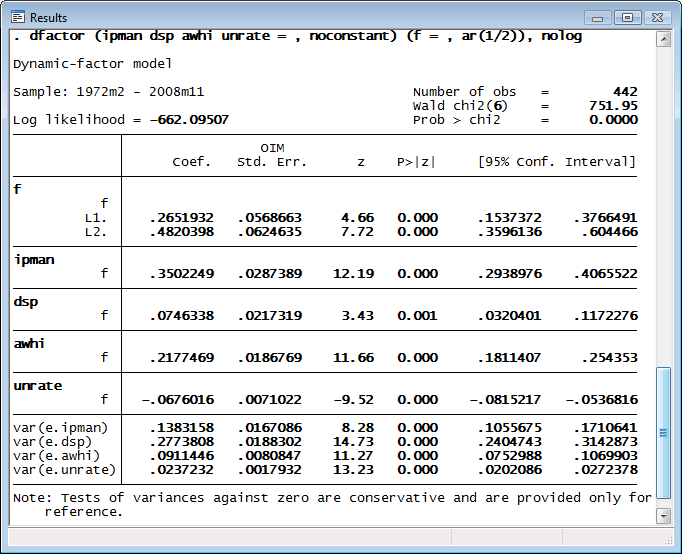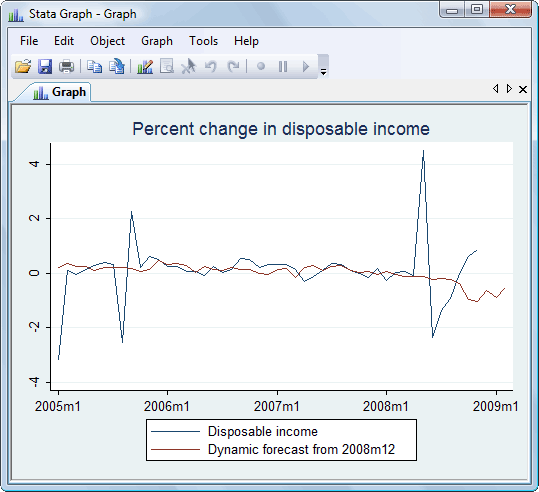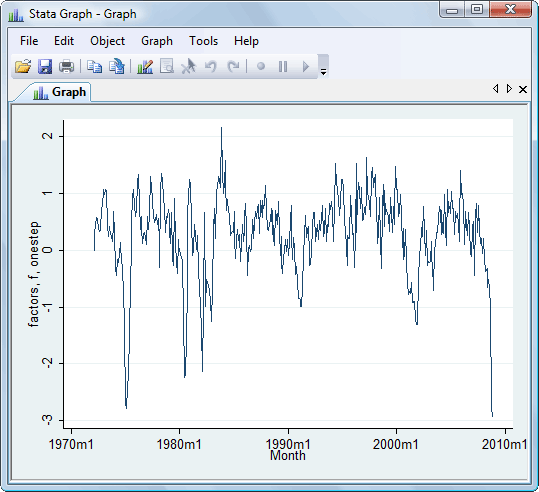»  Home »  Products »  Stata 11 »   Dynamic-factor models

## Dynamic-factor models

Stata’s new dfactor command estimates the parameters of dynamic-factor models by maximum likelihood. Dynamic-factor models are flexible models for multivariate time series in which the observed endogenous variables are linear functions of exogenous covariates and unobserved factors, which have a vector autoregressive structure. The unobserved factors may also be a function of exogenous covariates. The disturbances in the equations for the dependent variables may be autocorrelated.

We have data on industrial production (ipman), real disposable income (dsp), weekly hours worked (awhi), and the unemployment rate (unrate). We suspect there exists a latent factor that can explain all four of these series, and we conjecture that latent factor follows an AR(2) process.

The first step is to fit our model:With our model fit, let’s obtain dynamic forecasts for disposable income beginning in December 2008:

. tsappend, add(3)
. predict dsp_f, dynamic(tm(2008m12))
. tsline dsp dsp_f if month >= tm(2005m1)Even more interesting is the path of our unobserved factor. We have hypothesized that all our observed variables follow the unobserved latent factor. We can obtain the one-step predictions of the factor by typing

. predict factor, factor


We can then trace the path of the factor by graphing the result:

. tsline factorExtracting the latent factor in this manner is sometimes referred to as extracting or estimating an indicator.

dfactor also estimates the parameters of static-factor models, seemingly unrelated regression (SUR) models, and vector autoregressive (VAR) models by maximum likelihood. dfactor allows for constraints on the covariance matrix of the errors in an SUR model and a VAR model.

After estimation, you can predict both the endogenous variables and the unobserved factors. In addition to one-step predictions, dfactor can produce dynamic multistep predictions.

For a complete list of what’s new in time-series analysis, click here.